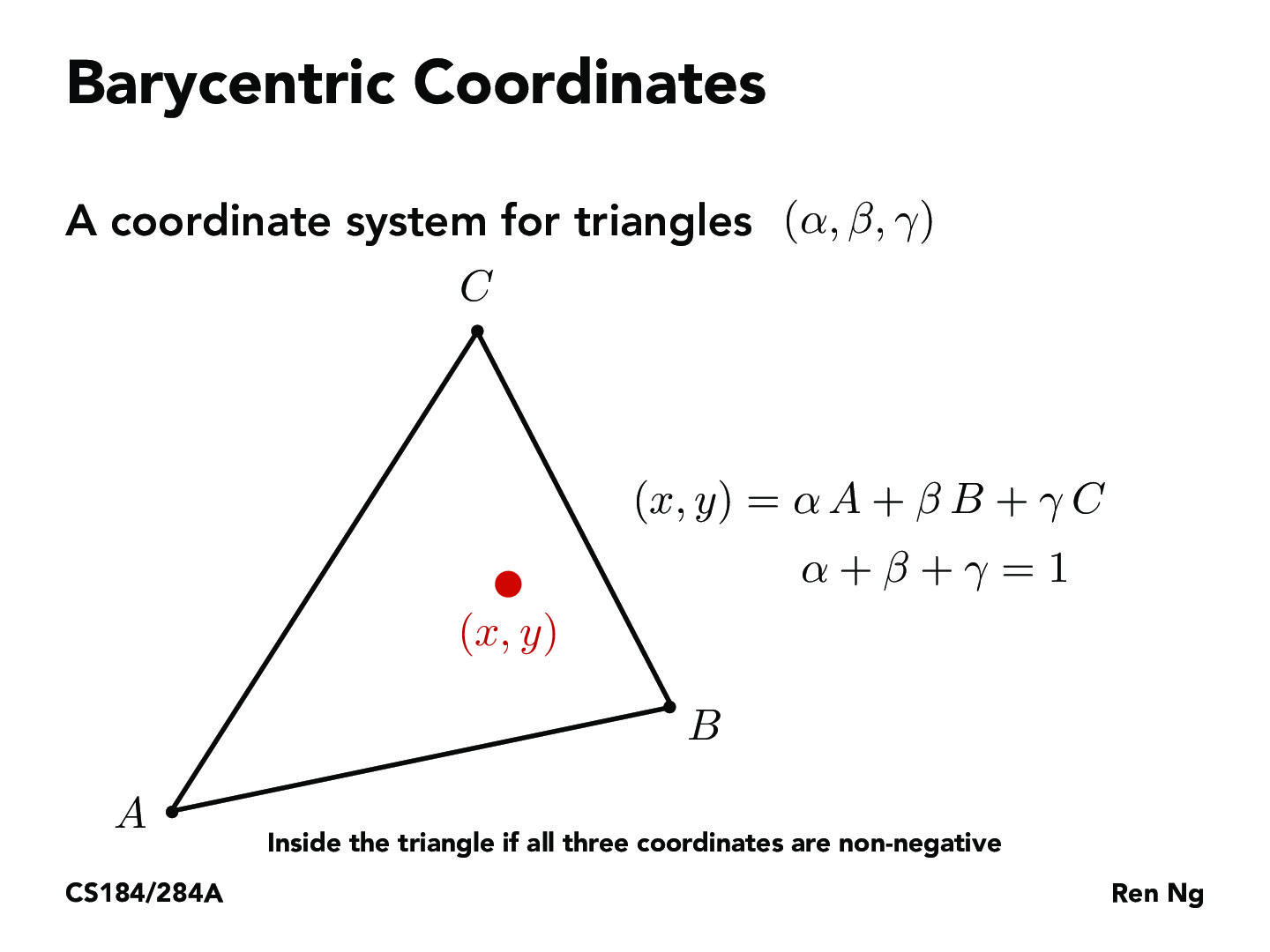You are viewing the course site for a past offering of this course. The current offering may be found here.Lecture 5: Texture Mapping (13)andrewyli

can we use these coordinates to evaluate whether x, y is inside the triangle based on the validity of alpha, beta, gamma, also maybe restricting them to be greater than 0?shariewang

^ yes! My comment was going to be about this. Barycentric coordinates, or areal coordinates, can extend outside the triangle, and in this case, one or more of the coordinates will be negative. Thus, as the slide says, the point is inside the triangle if all three coordinates are non-negative. Note that by derivation of these coordinates, there is one unique combination for each point.jcebreros1

I believe alpha+beta+gamma do not have to be equal to 1 for the point-in triangle-test. But what we have is that if alpha+beta+gamma=1 then they are unique. Does anyone know what advantage unique barycentric coordinates have over non-unique coordinates?leonardodtang

My guess would be that unique barycentric coordinates are essentially "normalized" in the sense that alpha+beta+gamma=1. The ratio between A, B, and C are unique given an (x, y) pair, so non-unique coordinates for alpha, beta, and gamma would just add up to some constant k and the new barycentric coordinates would just be kalpha, kbeta, and k*gamma. By using non-unique barycentric coordinates that don't add up to 1, it adds an extraneous k that doesn't really add any information about the system, instead necessitating us to keep track of variable k in future calculations. It's a similar argument as to why do we make a general habit of normalizing vectors in linear algebra applications. In the context of a real world example like signal filters, it's easier to make the "gain" scale from 0 to 1 since that makes the most physical sense, rather than using something like 0 to k and needing to factor k into all of our future calculations as opposed to 1.anthonyhsyu

Yes, the alpha+beta+gamma=1 is an additional constraint to make a unique solution. When solving for the system, we want to find alpha, beta, and gamma given just x and y, so we can only form two equations to solve for three variables. Adding this constraint adds a third equation to help find a unique solution.camrankolahdouz

^ I was wondering how it would be solve-able with the three unknowns and two equations. Thanks for the sanity checkandrewdotwang

Seems like if any of alpha, beta, gamma are less than 0, then the point is not inside the triangle, but still in the plane spanned by A, B, C.

[unaffiliated]

yup, you'll need to check that all of them are between 0 and 1 to be sure the point is in the triangle, and otherwise, it'd still be in the same plane.

You must be enrolled in the course to comment